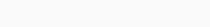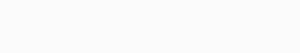# Unix time to datetime in Python

Epoch is the name of the day of 1st January 1970. Unix timestamp means the number of seconds that have passed since 1st January 1970(Epoch) till today and so on. This text will show the conversion of the Unix timestamp or Epoch to a readable datetime in Python programming. So, read the text below to learn and enjoy programming.

Install the datetime module using the following syntax.

`pip install datetime`

## Unix timestamp:

datetime.now()- This function is used to obtain the present datetime.
timestamp()- This method converts the present datetime to the Unix timestamp(Epoch).

This code is for printing a Unix timestamp in Python.

```# import the required module
from datetime import datetime
# function to get the Unix timestamp
Epoch=datetime.now().timestamp()
# print the Unix timestamp
print(Epoch)```
`Output:`These are the seconds that have passed from the day of 1st January 1970 to 24 November 2022(today’s date).

## Unix timestamp to readable datetime:

You will be able to convert the Unix timestamp to the readable datetime by using the following function in Python.

datetime.fromtimestamp()- Using this function you can convert the Unix timestamp to the readable datetime in Python. This function commands the Python interpreter to convert from Unix timestamp to the readable datetime.

This is the code that converts the Unix timestamp to the readable datetime in Python.

```# import the required module
from datetime import datetime
# function to get the Unix timestamp
Epoch=datetime.now().timestamp()
# print the Unix timestamp
print(Epoch)
#  conversion of the Unix timestamp to the readable datetime
`Output:`